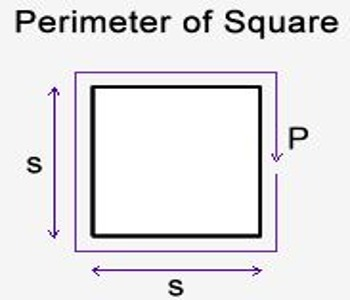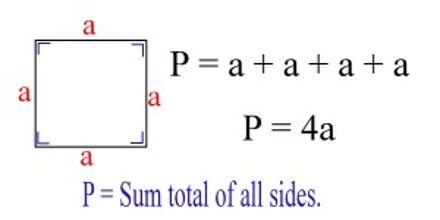Calculating the Perimeter of a Square

Perimeter of a Square means the total distance around the outside of a square. The perimeter of a square is the distance around the outside of the square. A square has four sides of equal length. The formula for finding the perimeter of a square is 4*(Length of a Side).I the case of a square, all four sides are the same length, so the perimeter is four times the length of a side. Or as a formula:

Perimeter = 4S

where; S is the length of any one side.

Given a square with side s, the perimeter (P) or distance around the outside of the square can be found by doing

P = a + a + a + a = 4 × aExample: Find the perimeter of a square shaped playground whose each side length 12 m.

We know a square has four equal sides. So, we can easily calculate the perimeter of a square.

The formula for finding the perimeter of a square is:

P = 4 × length of a side (S)

= 4 × 12 m = 48 m